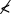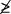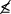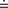## Common Math Symbols

The following symbols are commonly used in basic math and algebra. Be sure to know what each symbol represents.
• = is equal to
• ≠ is not equal to
• > is greater than
• < is less than
• ≥ is greater than or equal to (also written)
• ≤ is less than or equal to (also written)
•is not greater than
•is not less than

•is not greater than or equal to

•is not less than or equal to

• ≈ is approximately equal to (also written)